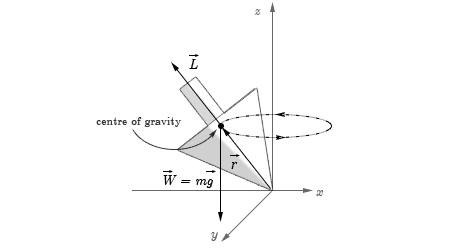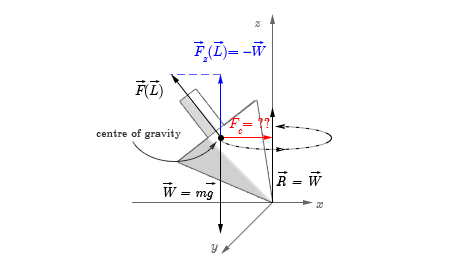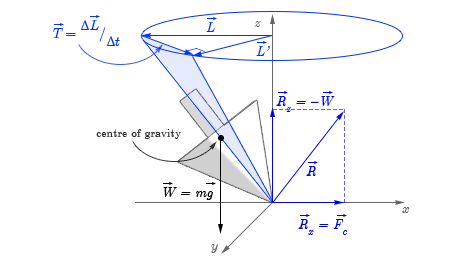# Gyroscopic precession: What provides the centripetal force?

• H Smith 94
In summary, the conversation discusses the explanation for the precession of a spinning top when it is tilted away from its axis of symmetry. The torque produced by the weight and reaction force causes the precession, but the location of the centripetal force is still unclear. The conversation delves into the different components and forces involved, including the horizontal component of the reaction force and the role of friction. Ultimately, it is determined that the precession is caused by the torque forcing a change in angular momentum, while the centripetal force is determined by the surface at the contact point.

#### H Smith 94

Gold Member
Hi there!

I've been searching the internet for hours trying to find a decent explanation to this question.

I'm thinking particularly of the example of a precessing spinning top.(Note: I own this diagram. Please do not use it without direct and written permission.)
So when the top is tilted away from the axis of symmetry, its weight W and the reaction force produce a couple set apart by the distance r from the point of contact and the centre of mass/gravity. The best explanation I've found is that the torque T produced by this couple produces precession, wherein

T = r × W
However this does not seem to explain where the centripetal force occurs because - according to the vectorial product - this torque acts in the direction of angular velocity, not perpendicular to it! In the above diagram the torque would be pointing towards the viewer from the centre of gravity, right?

Harry

Last edited:
Well, keep in mind that half of the top, the one that is rotating towards the vertical axis, is sort of "whipping" the top towards that axis. While of course the counter-argument would be "well, the other half whips away from it", I think the precessing movement of the top kinda causes the toward-whipping to be slightly greater than the away-whipping, thus producing a net centripetal force.
Imagine the extreme case where the top is precessing almost horizontally, and precessing with high speed. In that case, the part of the top rotating away from the vertical axis would almost be still in relation to the ground, whereas the part rotating towards the vertical axis would be almost double the speed because it adds the precessing speed to the rotation speed. Sort of like a gravity assist that space vessels do: http://upload.wikimedia.org/wikiped...ot.svg/2000px-Gravitational_slingshot.svg.png

Last edited:
H Smith 94 said:
In the above diagram the torque would be pointing towards the viewer from the centre of gravity, right?
Yes, that is correct.

H Smith 94 said:
However this does not seem to explain where the centripetal force occurs because - according to the vectorial product - this torque acts in the direction of angular velocity, not perpendicular to it!
In the diagram above the angular velocity is almost entirely in the direction of L, which is perpendicular to the torque. The small angular velocity in the z direction is deliberately neglected.

DaleSpam said:
In the diagram above the angular velocity is almost entirely in the direction of L, which is perpendicular to the torque. The small angular velocity in the z direction is deliberately neglected.
So the z-component of the angular momentum Lz produces a force in the z-direction to balance out the weight W, in turn creating a net vertical force of zero. Would this be a correct adaptation to the diagram? (Note that I have removed all vectors except forces).(Please do not use diagram without direct and written permission.)
Adaptations in blue, further unknowns in red. Here, F(L) is intended to represent the force due to the angular momentum vector L, and Fz(L) its z-component.
(Update: Diagram should show, R = −W)

Is it the product of the squared angular momentum L and the negative radius dx of the path traced out by the centre of mass?

Fc = ma = m (−|L|2 dx)
I'm sorry if I'm being dense but this question has perplexed me for a while!

Harry

Last edited:
OK, so I would do this problem, not rotating about the center of gravity, but rotating about the contact point at the origin.

There are two forces acting on the top. One is the weight, and the other is the reaction force at the origin. The reaction force has two components, a vertical component which cancels out the weight, and a horizontal component which causes the centripetal motion of the center of mass. The horizontal component depends on the surface at the contact point, and could go to zero in the case of a frictionless surface.

Neither of those two components exert a torque about the contact point, so the only torque is due to the weight. As discussed earlier that causes a change in angular momentum out of the plane of the drawing. Using the usual gyroscope approximation that the angular momentum is entirely along the axis of the gyroscope, this causes the gyroscope to precess.

If there is no friction then the contact point will slide around in a circle while the center of mass remains stationary. So the torque from the weight determines the precession rate, but whether or not that precession is associated with any centripetal motion is determined by the horizontal component of the force at the contact point.

•gregorspv
DaleSpam said:
"There are two forces acting on the top. One is the weight, and the other is the reaction force at the origin. The reaction force has two components, a vertical component which cancels out the weight, and a horizontal component which causes the centripetal motion of the center of mass. The horizontal component depends on the surface at the contact point, and could go to zero in the case of a frictionless surface."

So essentially, the friction force stops the gyroscope from slipping at the point of contact and rotating about the centre of mass? Okay, that seems to make sense! I can imagine spinning a top on ice and, from what I can imagine, it would just fall over and spin about on the floor.

DaleSpam said:
"Neither of those two components exert a torque about the contact point, so the only torque is due to the weight. As discussed earlier that causes a change in angular momentum out of the plane of the drawing. Using the usual gyroscope approximation that the angular momentum is entirely along the axis of the gyroscope, this causes the gyroscope to precess. "

I see, so the precession motion of the gyroscope is due to the torque essentially forcing the angular momentum to constantly change in a forward rotation.

I've again updated my diagram to reflect this.(Please do not use diagram without direct and written permission.)
Thank you so much for your help, Dale!

Best regards,

Harry

H Smith 94 said:
I can imagine spinning a top on ice and, from what I can imagine, it would just fall over and spin about on the floor.
Why would it just fall over, without friction? The tilted spin axis could still precess around a vertical axis, that goes through the center of mass, instead through the contact point.

A.T. said:
Why would it just fall over, without friction? The tilted spin axis could still precess around a vertical axis, that goes through the center of mass, instead through the contact point.

I meant it would no longer rotate about an axis displaced from the centre of mass, hence no longer obeying the parallel axis theorem. I don't know why I used that exact wording and, yes, I agree it was horrendously ambiguous!

Sounds like you got it. Glad to help.

The only thing that I would correct is that on the diagram L goes along the axis of the gyroscope. So diagonally up and to the left in the diagram, not horizontally to the left.

DaleSpam said:
Sounds like you got it. Glad to help.

The only thing that I would correct is that on the diagram L goes along the axis of the gyroscope. So diagonally up and to the left in the diagram, not horizontally to the left.

Ah yes! I've recified it in the final diagram to show L - Lz and L' - Lz (so the L vector is acting in only the x-y plane). This way ΔL = (L' - Lz) - (L - Lz) = L' - L.

Thank you again!

Harry

## 1. What is gyroscopic precession?

Gyroscopic precession is the phenomenon where an object's axis of rotation changes in response to an external force. This is due to the conservation of angular momentum.

## 2. How does gyroscopic precession work?

Gyroscopic precession works by the application of an external force, such as a torque, on an object with a spinning axis. This force causes the object's axis of rotation to change direction, resulting in precession.

## 3. What provides the centripetal force in gyroscopic precession?

The centripetal force in gyroscopic precession is provided by the torque applied to the spinning object. This torque causes the object's axis of rotation to change direction, resulting in precession.

## 4. What are some real-world applications of gyroscopic precession?

Gyroscopic precession has many practical applications, such as the stability of bicycles, gyroscopes used in navigation systems, and the gyroscopic effect in sports equipment like tennis rackets and golf clubs.

## 5. How is gyroscopic precession related to angular momentum?

Gyroscopic precession is related to angular momentum through the conservation of angular momentum. This means that the total angular momentum of a system remains constant, and any changes in the direction of rotation are due to external forces.